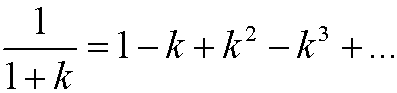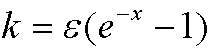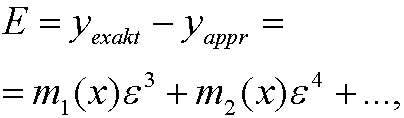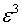# 5. Comparison with the exact solution.

Again consider the differential equationAs we said before, the exact solution is thenBy using maclaurin expansion we know thatfor small values of k. If we insertwe getNow compare with the approximate solution from last partWe see that the error E in the approximation thus isfor some dunctions m1(x), m2(x),… (that we can compute!). We say that the error E is of orderand write E=O(), where O stands for big ordo.